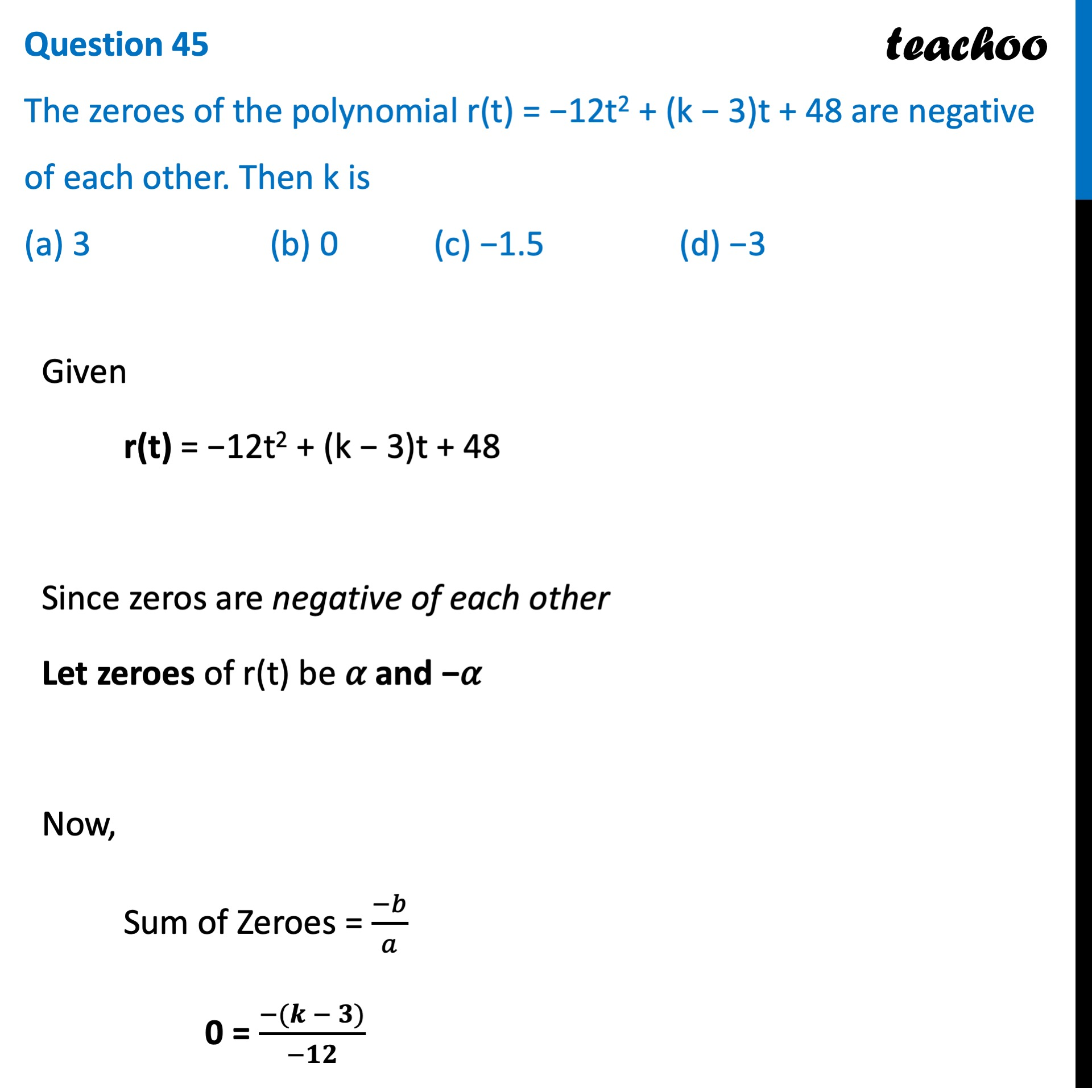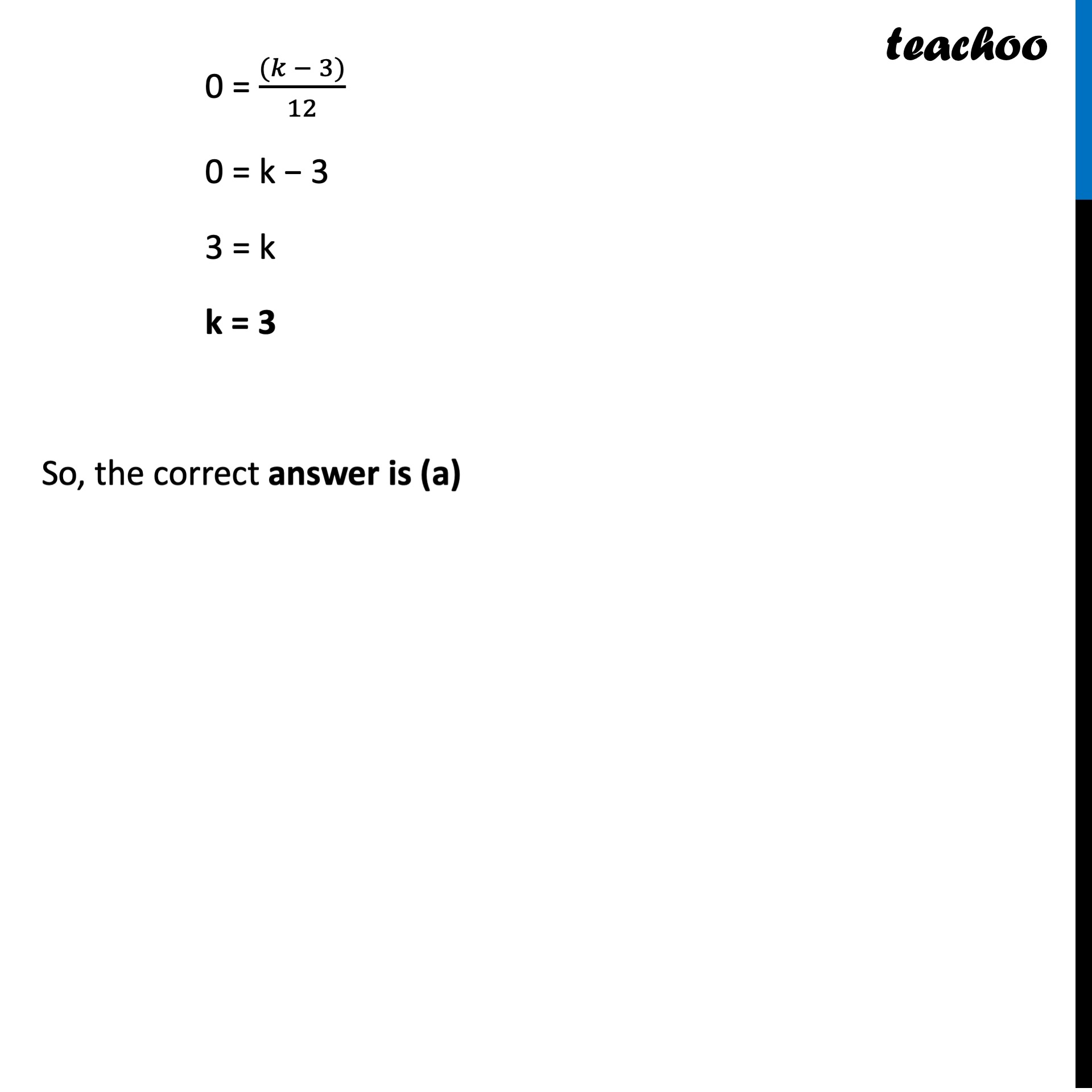## (a) 3   (b) 0   (c) −1.5   (d) −31. Class 10
2. Solutions of Sample Papers for Class 10 Boards
3. CBSE Class 10 Sample Paper for 2022 Boards - Maths Standard [MCQ]

Transcript

Question 45 The zeroes of the polynomial r(t) = −12t2 + (k − 3)t + 48 are negative of each other. Then k is (a) 3 (b) 0 (c) −1.5 (d) −3 Given r(t) = −12t2 + (k − 3)t + 48 Since zeros are negative of each other Let zeroes of r(t) be 𝛼 and −𝛼 Now, Sum of Zeroes = (−𝑏)/𝑎 0 = (−(𝒌 − 𝟑))/(−𝟏𝟐) 0 = ((𝑘 − 3))/12 0 = k − 3 3 = k k = 3 So, the correct answer is (a)